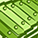# App Development

cancel
Showing results for
Did you mean:Contributor III

## year aggregation different from sum of month aggregation

Hi everybody, in the same graph, I can aggregate data by Year/Quarter/Month

And year aggregation is different from sum of month aggregation.

After investigating, the problem comes from the formula in the graph, to simplify,
let's say : formula=sum(a)/sum(b).
So for the first quarter for exemple, the automatic sum for the quarter will be equivalent to (a1+a2+a3)/(b1+b2+b3) which is different from the sum of the 3 first months : (a1/b1)+(a2/b2)+(a3/b3).

Hope my question is clear enough.
Is there a way to solve this problem ? Any suggestions ?

Labels (1)
• ### time aggregationPartner

Hi there!